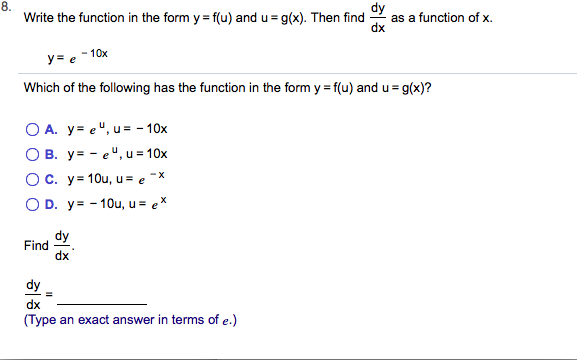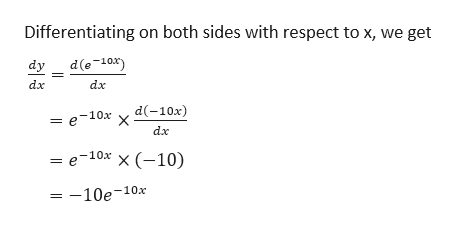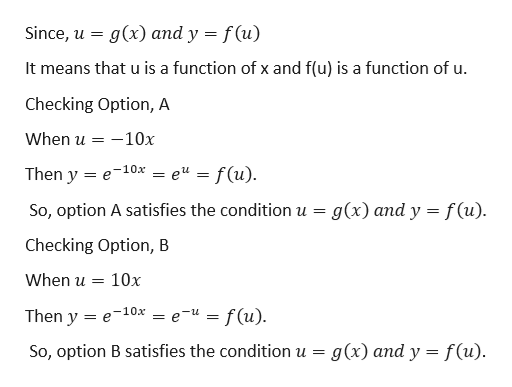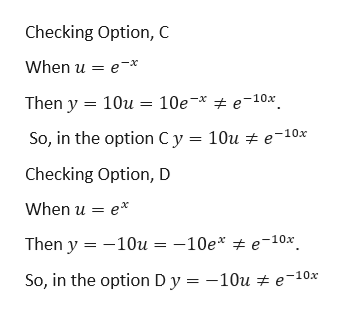# 8.Write the function in the form y = f(u) and u g(x). Then findas a function of xdxye10xWhich of the following has the function in the form y f(u) and u g(x)?O A. ye,u= -10xO B. y-e, u 10x=O C. y 10u, u = e-XO D. y-10u, u e*dyFinddxdydx(Type an exact answer in terms of e.)

Question
27 views

Can you help me step by step with this problem?help_outlineImage Transcriptionclose8. Write the function in the form y = f(u) and u g(x). Then find as a function of x dx ye10x Which of the following has the function in the form y f(u) and u g(x)? O A. ye,u= -10x O B. y-e, u 10x = O C. y 10u, u = e -X O D. y-10u, u e* dy Find dx dy dx (Type an exact answer in terms of e.) fullscreen
check_circle

Step 1

Given,help_outlineImage TranscriptioncloseDifferentiating on both sides with respect to x, we get d(e-10x) dy dx dx dx = e-10x x (-10) -10e-10x fullscreen
Step 2

Now,help_outlineImage Transcriptionclosef (u) = g(x) and y Since, u It means that u is a function of x and f(u) is a function of u Checking Option, A When u 10x = Then y e10x = e" = f(u) g(x) and y f(u) So, option A satisfies the condition u Checking Option, B When u = 10x Then y e10x = e-" = f(u). g(x) and y f(u). So, option B satisfies the condition u = fullscreen
Step 3

Again

...help_outlineImage TranscriptioncloseChecking Option, C When u ex Then y e-10x. 10u = 10e* So, in the option Cy 10u # e-10x Checking Option, D When u e e-10x Then y 10u = -10e* So, in the option Dy = -10u e-10x fullscreen

### Want to see the full answer?

See Solution

#### Want to see this answer and more?

Solutions are written by subject experts who are available 24/7. Questions are typically answered within 1 hour.*

See Solution
*Response times may vary by subject and question.
Tagged in